### Sawtooth Genetic Algorithm (SawtoothGA)## Introduction

xlOptimizer implements SawtoothGA, a variant of Genetic Algorithms (GA) that uses variable population size and partial re-initialization of the population. It was introduced by Koumousis and Katsaras .

## How does it work?

According to SawtoothGA, the population size follows a predefined scheme which is characterized by mean population M, amplitude of variation D and period of variation T. The population size is decreased linearly during each period of variation. At the end of the period, the size is restored to its original value by the addition of randomly generated individuals.

From parametric studies with a large test-bed of unimodal and multimodal problems, it became evident that strong re-initialization of the population, i.e. large values of D/M , is beneficial.

## Mathematical formulation

The mathematical formulation is similar to the one described for Genetic Algorithms. The sawtooth variation scheme is described by the following picture: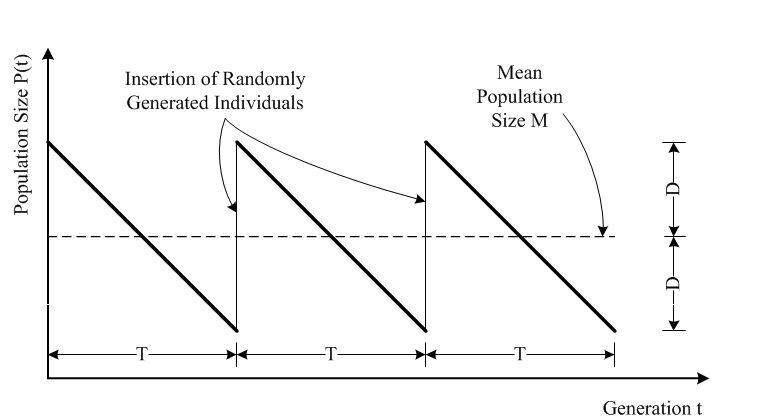## Options in xlOptimizer

The following options are available in xlOptimizer:

#### Step 1Enter a short title for the scenario. The log files will be saved to a subfolder with this name.

#### Step 2Select the parameters of SawtoothGA for the variation of population. The optimum values depend on the difficulty of the problem. Usual settings are Μ=50, D=45, T=10. This means that the mean value is 50 individuals, the amplitude of variation is 45, i.e. the population varies from 50+45=95 individuals to 50-45=5 individuals, and the period of variation is 10 generations.

#### Step 3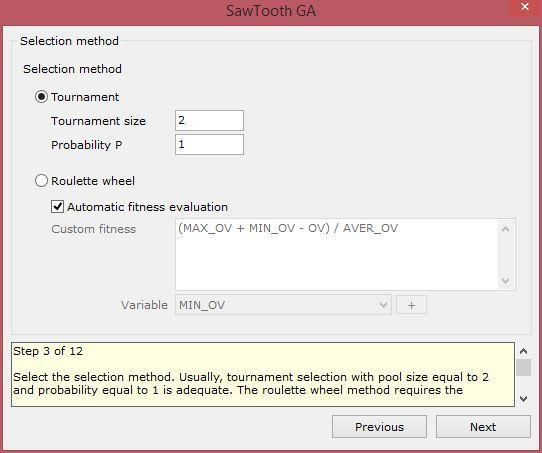Choose the selection method. The method can be either tournament selection or biased roulette wheel. Usually, tournament selection with pool size equal to 2 and probability equal to 1 is adequate. The roulette wheel method requires the definition of a fitness function, based on the objective function. The evaluation of the fitness can be performed automatically, or based on custom code. In the latter case, the variables that can be used are the following:

• MIN_OV: the minimum objective value within the members of the population.
• MAX_OV: the maximum objective value within the members of the population.
• AVER_OV: the average objective value within the members of the population.
• BEST_OV: the best objective value within the members of the population.
• WORST_OV: the worst objective value within the members of the population.
• OV: the objective value of the current individual for which the fitness is being evaluated.
• TARGET: the target objective value for target - problems.

The automatic evaluation is based on the following code:

• '(OV - MIN_OV) / AVER_OV' for maximization problems,
• '(MAX_OV + MIN_OV - OV) / AVER_OV' for minimization problems,
• '(ABS(MAX_OV - TARGET) + ABS(MIN_OV - TARGET) - ABS(OV - TARGET) / ABS(AVER_OV - TARGET)' for target-value problems.

Based on the above, a first level of normalization is achieved with the division by the average objective value.

#### Step 4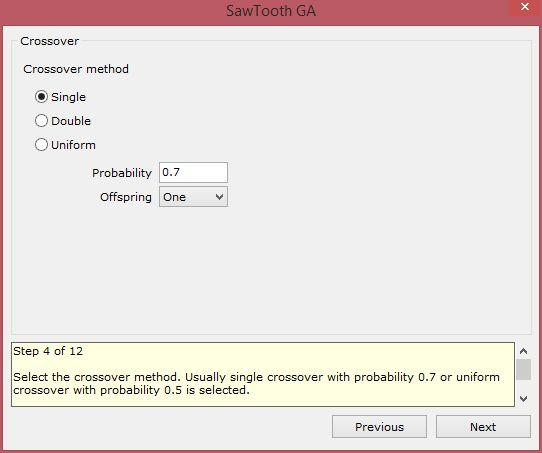Select the crossover method. Usually single crossover with probability 0.7 or uniform crossover with probability 0.5 is selected. Also, choose whether the selected pair of chromosomes will produce one or two offspring.

#### Step 5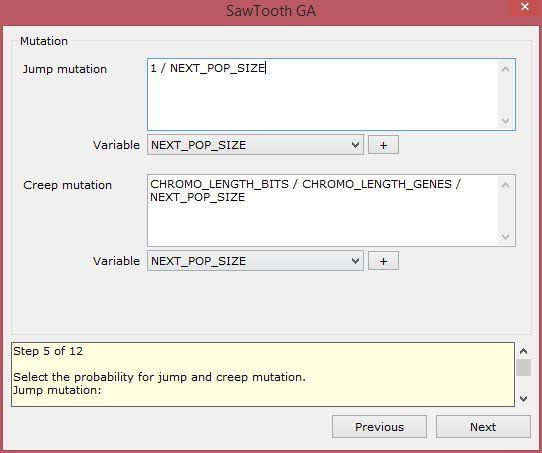Select the probability for jump and creep mutation.

• Common setting for jump mutation code is '1 / NEXT_POP_SIZE'. In this case the random number generator is invoked NEXT_POP_SIZE * CHROMO_LENGTH_BITS times.
• Common setting for creep mutation code is 'CHROMO_LENGTH_BITS / CHROMO_LENGTH_GENES / NEXT_POP_SIZE'. In this case the random number generator is invoked NEXT_POP_SIZE * CHROMO_LENGTH_GENES times.

#### Step 6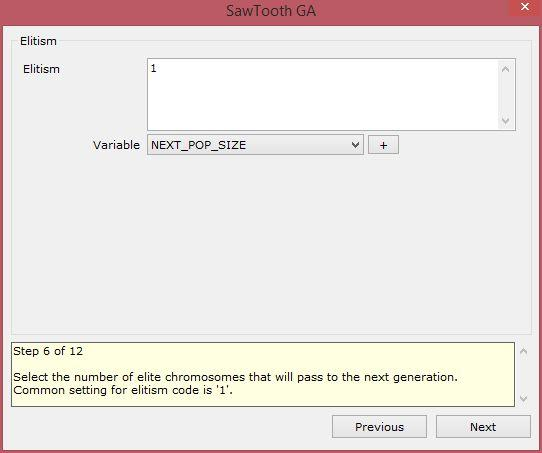Select the number of elite chromosomes that will pass to the next generation. Common setting for elitism code is '1', the single best individual is transferred to the next generation.

#### Step 7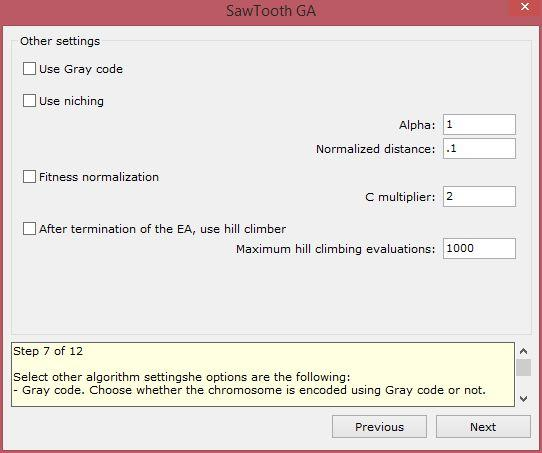Select other algorithm settings. The options are the following:

• Gray code: Choose whether the chromosome is encoded using Gray code or not.
• Niching: Select the alpha coefficient (power law exponent for sharing function; typically = 1.0) and the normalized distance ( 1/normalized distance can be viewed as the number of regions over which the sharing function should focus). This affects the design variables that have been set to participate in a niching strategy.
• Hill climber: Choose whether after the termination of the GA a local optimizer, namely steepest ascend hill climber, is invoked. Also select the maximum number of function evaluations for the local optimizer.

#### Step 8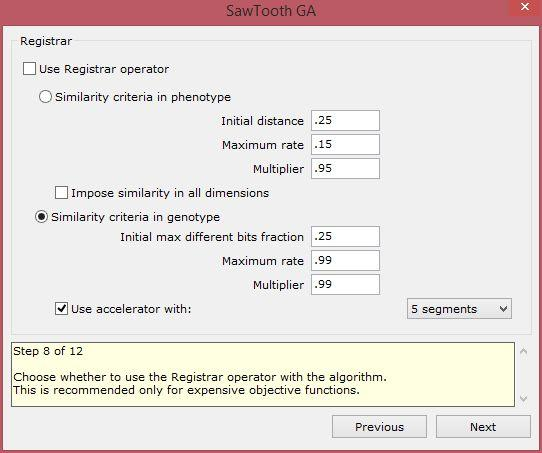Choose whether to use the Registrar operator with the algorithm. This is recommended only for expensive objective functions.

#### Step 9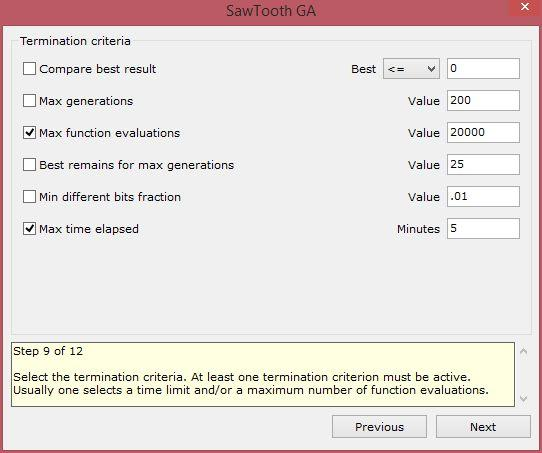Select the termination criteria. At least one termination criterion must be active. Usually one selects a maximum number of function evaluations and/or a time limit.

#### Step 10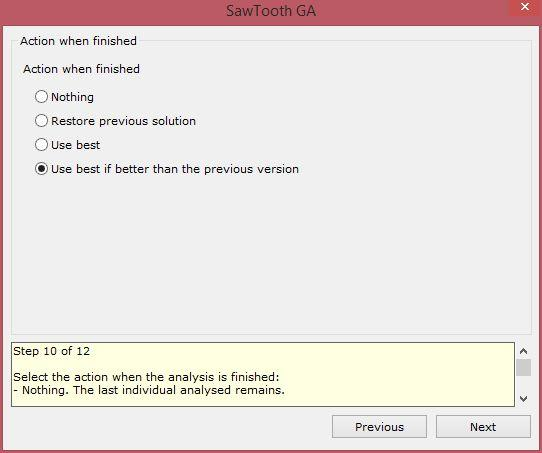Select the action when the analysis is finished:

• Nothing: The last individual analysed remains.
• Restore previous solution: The solution that was visible before the analysis is restored.
• Use best: The best solution that was discovered during the analysis is applied.
• Use best if better than the previous version (recommended): The best solution that was discovered during the analysis is applied only if it is better than the previous solution. In the opposite case the previous solution is restored.

#### Step 11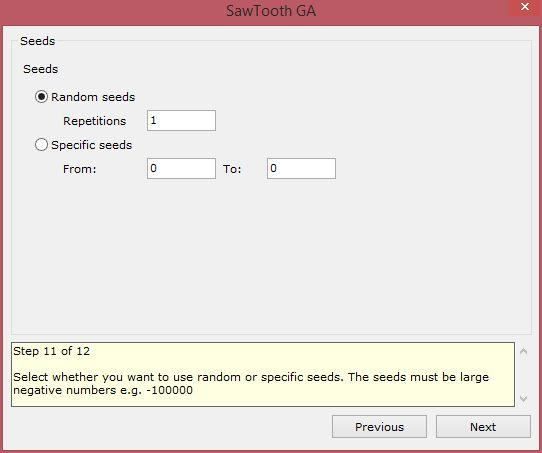Select whether you want to use random or specific seeds for the random number generator. The seeds must be large negative numbers e.g. -100000. Usually a number of analyses with random seeds is performed. The filename of the log is the seed used in the specific analysis.

#### Step 12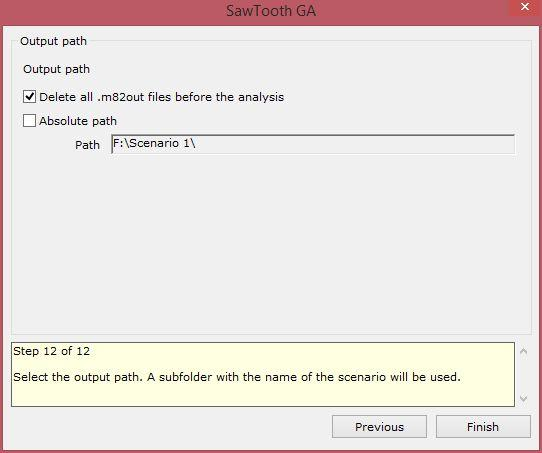Select the output path. A subfolder with the name of the scenario will be used. Usually a relative path is used i.e. the absolute path check box is not checked. This results in the output logs being saved in the same path as the project file.